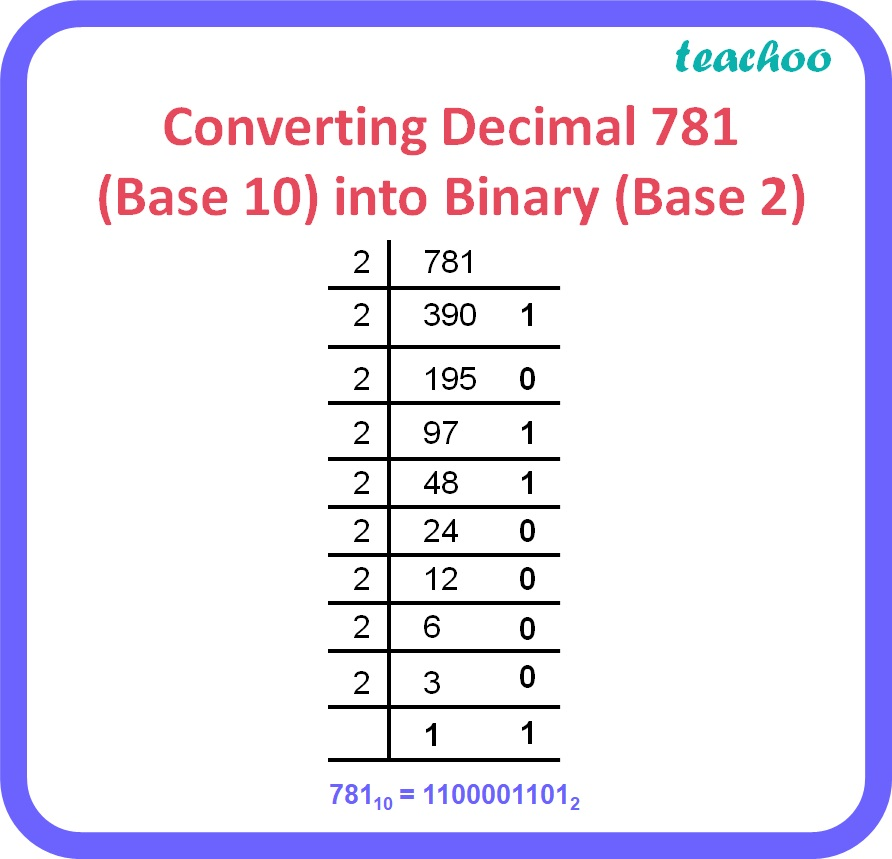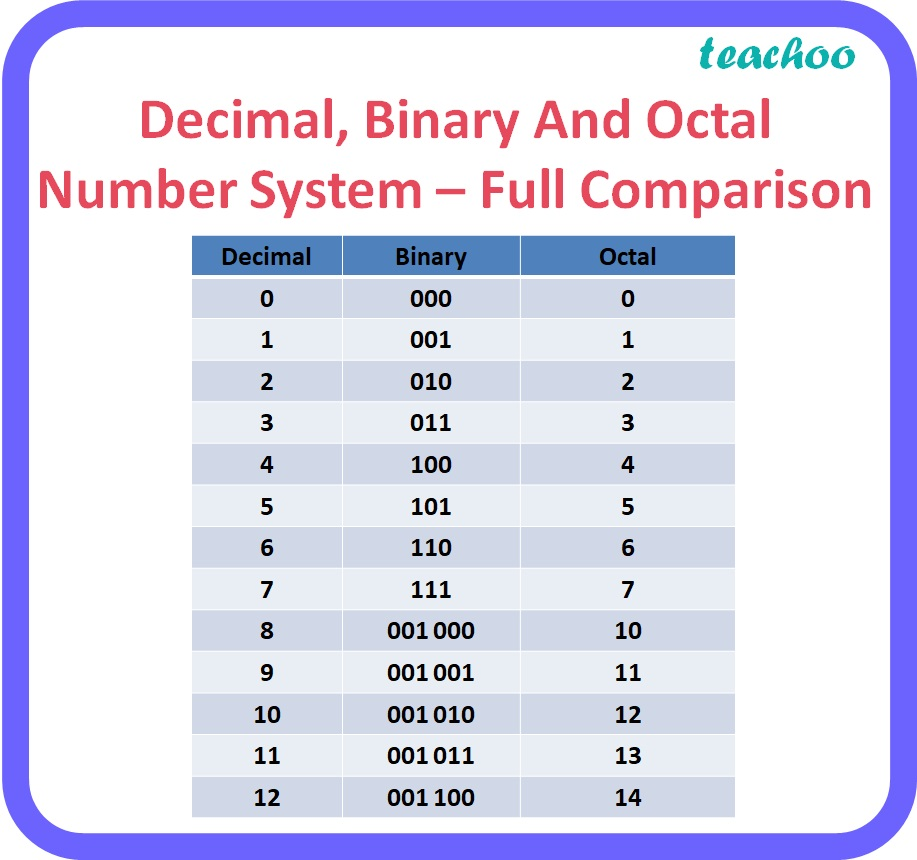5 Mark Questions

Computer Science - Class 11
Chapter 2 Class 11 - Encoding Schemes and Number System

## (c) Convert Octal number 321.7 info its Binary equivalent

### (a) 781 10 to its binary equivalentTherefore, 781 10 = 1100001101 2

Explanation:

• Repeatedly divide the decimal number by 2 until the quotient becomes 1 and record all the remainders.
• The remainders should be written bottom to upwards to get the binary equivalent of the decimal number.

### (b) 101101.001 2 to its decimal equivalent

101101.001 = 1 x 2 5 + 0 x 2 4 + 1 x 2 3 + 1 x 2 2 + 0 x 2 1 + 1 x 2 0 + 0 x 2 -1 + 0 x 2 -2 + 1 x 2 -3

= 32 + 0 + 8 + 4 + 0 + 1 + 0 + 0 + 0.125

= 45.125 10

Explanation:

• To obtain the decimal equivalent of a binary number, individual digits of binary number should be multiplied by powers of 2 starting with the rightmost digit multiplied by 2 0 , second last digit multiplied by 2 1 , third last digit multiplied by 2 2 and so on up to the leftmost digit.
• For digits after the decimal point, the leftmost digit should be multiplied by 2 -1 and the next digit by 2 -2 and so on up to the rightmost digit.

### (c)  321.7 8 to its binary equivalent

 3 2 1 7 011 010 001 111

Therefore, 321.7 8 = 11010001.111 2

Explanation:

• To obtain binary equivalent of an octal number, individual digits of octal number should be converted to binary in groups of three digits as given in the table below.Get live Maths 1-on-1 Classs - Class 6 to 12

### Transcript

Converting Decimal 781 (Base 10) into Binary (Base 2) Decimal, Binary And Octal Number System – Full Comparison